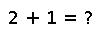# eigvals

eigvals(A,[irange,][vl,][vu]) -> values

Returns the eigenvalues of `A`. If `A` is `Symmetric`, `Hermitian` or `SymTridiagonal`, it is possible to calculate only a subset of the eigenvalues by specifying either a `UnitRange` `irange` covering indices of the sorted eigenvalues, or a pair `vl` and `vu` for the lower and upper boundaries of the eigenvalues.

For general non-symmetric matrices it is possible to specify how the matrix is balanced before the eigenvector calculation. The option `permute=true` permutes the matrix to become closer to upper triangular, and `scale=true` scales the matrix by its diagonal elements to make rows and columns moreequal in norm. The default is `true` for both options.

## Examples

``````julia> eigvals(10) - 6
1-element Array{Float64,1}:
4.0``````

Checking you are not a robot: